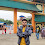# HackerRank Polar Coordinates solution in python

In this HackerRank Polar Coordinates problem solution in python, Polar coordinates are an alternative way of representing Cartesian coordinates or Complex Numbers.

You are given a complex z. Your task is to convert it to polar coordinates.

## Problem solution in Python 2 programming.

```from math import atan2

z = complex(input())
x,y = z.real, z.imag
r = (x**2+y**2)**.5
phi = atan2(y,x)

print r
print phi```

## Problem solution in Python 3 programming.

```# Enter your code here. Read input from STDIN. Print output to STDOUT
import cmath
print(*cmath.polar(complex(input())), sep='\n')```

### Problem solution in pypy programming.

```# Enter your code here. Read input from STDIN. Print output to STDOUT
from cmath import phase

inp = raw_input()

print round(abs(complex(inp)),3)
print round(phase(complex(inp)),3)```

### Problem solution in pypy3 programming.

```import cmath

c = complex(input())
print(abs(c))
print(cmath.phase(c))```

1.1.